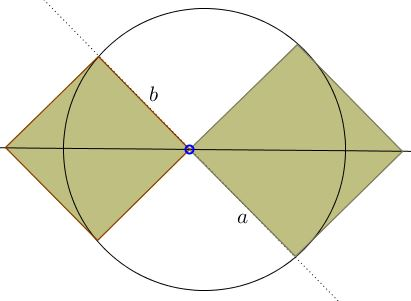# Sum of Two Squares

2 March, 2016, Created with GeoGebra

### Problem

A chord that crosses a diameter of a circle at a $45^{\circ}\;$ angle is split by the diameter into two segments of lengths $a\;$ and $b.$Prove that $a^2+b^2\;$ is independent of the position of the chord.

### Proof

Form two squares on the segments $a\;$ and $b\;$ for which the diameter in question makes the diagonals and consider the right triangle formed by the sides of these squares that lie on the same side of the diameter.The vertex housing he right angle in the triangle lies on the diameter. Rotate the triangle $90^{\circ},\;$ $180^{\circ},\;$ and $270^{\circ}\;$ around the center of the circle. The four right vertices form a square and lie two-by-two on two perpendicular diameters, so that the four triangles fit snugly to form a square (with a square hole) with the side length equal to $\sqrt{a^2+b^2}\;$ and area equal to $a^2+b^2.\;$ But all squares inscribed into a fixed circle are congruent and thus have equal areas.

### Extra

Daniel Liu has observed that there is a one line proof based on the observation that the hypotenuse of the triangle we just used is a chord in the circle that subtends a central angle of $90^{\circ}.\;$ This is due to the symmetry in the diameter and the property of the angle formed by two secants (the sides of the two squares here). Thus it is of fixed length.

### References

1. R. Nelsen, Proofs Without Words III, MAA, 2016, p. 35